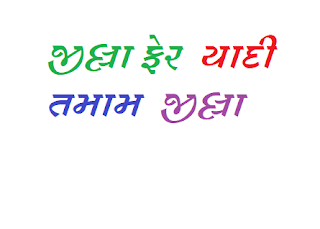## 20 February 2016

### Get Speed in Maths of Competitive Exam By Short Tricks...

Trick-1..

Trick of number 9

Take the nine digits in order and remove the 8: 12345679.
Then multiply by 9:
12345679*9 = 111111111

now tries multiplication by the multiples of 9:

12345679*18 = 222222222
12345679*27 = 333333333
12345679*36 = 444444444
12345679*45 = 555555555
12345679*54 = 666666666
12345679*63 = 777777777
12345679*72 = 888888888
12345679*81 = 999999999

Trick-2..

Trick for Calculate Reminder On Dividing The Number By 27 And 37

Consider number 34568276, we have to calculate the reminder on dividing this number by 27 and 37 respectively.
make triplets as written below starting from units place
34.........568..........276
now sum of all triplets = 34+568+276 = 878
divide it by 27 we get reminder as 14
divide it by 37 we get reminder as 27

For Example,

other examples for the clarification of the rule
let the number is 2387850765
triplets are 2...387...850...765
sum of the triplets = 2+387+850+765 = 2004
on revising the steps we get
2......004
sum = 6
divide it by 27 we get reminder as 6
divide it by 37 we get reminder as 6

Trick-3..

Trick for Calculate Reminder On Dividing The Number By 3

Method: - first calculate the digit sum, then divide it by 3, the reminder in this case will be the required reminder

For example: - 1342568
let the number is as written above
its digit sum = 29 = 11 = 2
so reminder will be 2

For Example,

34259677858
digit sum of the number is 64 = 10 = 1
reminder is 1

Like this get more short tricks of maths and score in the competitive exam with kachhua.com...

Kachhua.com can help you to prepare well in competitive exam by providing online material , video lectures and regular online tests(provided with Auto result and Daily solution)...

Now do online preparation of RRB Railway, GSRTC and other competitive  Exams with kachhua.com...
Crack the railway, GSRTC and other competitive exams by these online courses. Kachhua.com can help you to prepare well in railway, GSRTC and other competitive exams...
Click below links, if you want to pass the RRB railway, GSRTC or any other competitive exams easily...

click the below link for preparation of Mathematics with kachhua..